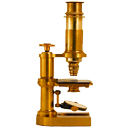# BiomatThe content of this page has not been vetted since shifting away from MediaWiki. If you’d like to help, check out the how to help guide!Name Biomat Software ImageJ Author Jiří Janáček Maintainer Jiří Janáček Source on GitHub Status

## Plugins for 3D image preprocessing.

Stack Linear Contrast - multiplies images in stack by coefficient obtained by linear interpolation of the “first” and “last” coefficient. A simple tool for compensation of contrast decreasing with depth within thick sample.

Lipschitz 3D Filter - top hat - subtracts slowly varying background calculated as lower Lipschitz envelope from the image.

Preprocessing example: Image of capillaries in brain

• PluginsBiomatStack Linear Contrast with parameters (multiplyers) “first”= 1.0 and “last”= 3.0
• ProcessFiltersGaussian blur 3D with “sigma”= 1 pixel
• PluginsBiomatLipschitz 3D Filter with parameter “slope”= 2 / pixel

## Plugins for detection of fibres in 3D image.

Tensor Line 3D Filter - enhances white fibers of uniform width sparsely distributed on dark background.

• PluginsBiomatTensor Line 3D Filter with “sigma” set to 3 pixels
• ImageAdjustBrightness/Contrast
• ProcessFiltersGaussian blur 3D with “sigma”= 1 pixel

Vector Line 3D Filter - enhances white fibers of varying thickness. Crossection of the fibers need not be circular. Parameter “sigma” in pixels corresponds to the largest diameter.

• PluginsBiomatVector Line 3D Filter with parameters “sigma”= 4 pixels, “scale number”= 2

## Plugins for evaluation of 2D images using heat equation.

2D Tensor Color Coding - standard color coding of 2D tensor image. Symmetric tensor is coded as channels of 32 bit image stacks.

2D Heat Kernel Tensor - second order moments of heat kernel calculated from 8 bit binary image.

2D Tensor Statistics - summary of tensor image (in ROI). “Value” is average trace of the tensor, “shape” is the ratio of its eigenvalues and “angle” of the first eigenvector is measured counterclockwise from the horizontal axis.